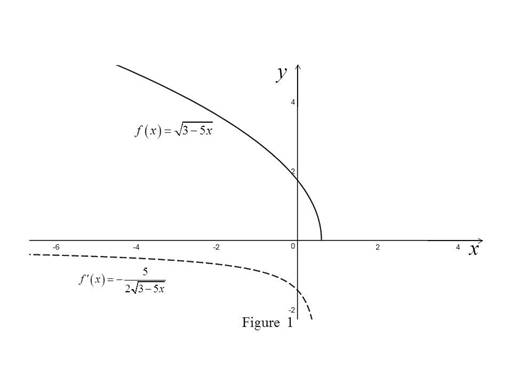# The derivative of the function f ( x ) = 3 − 5 x .### Single Variable Calculus: Concepts...

4th Edition
James Stewart
Publisher: Cengage Learning
ISBN: 9781337687805### Single Variable Calculus: Concepts...

4th Edition
James Stewart
Publisher: Cengage Learning
ISBN: 9781337687805

#### Solutions

Chapter 2, Problem 37RE

(a)

To determine

## To find: The derivative of the function f(x)=3−5x.

Expert Solution

The derivative of f(x) is f(x)=5235x.

### Explanation of Solution

Formula used:

The derivative of a function f, denoted by f(x), is

f(x)=limh0f(x+h)f(x)h (1)

Calculation:

Obtain the derivative of the function f(x).

Compute f(x) by using the equation (1),

f(x)=limh0f(x+h)f(x)h=limh035(x+h)35xh

Multiply and divide 35(x+h)+35x in the above expression

f(x)=limh035(x+h)35xh×35(x+h)+35x35(x+h)+35x=limh0(35(x+h))2(35x)2h{35(x+h)+35x}=limh035(x+h)(35x)h{35(x+h)+35x}=limh05hh{35(x+h)+35x}

Since the limit h approaches zero but not equal to zero, cancel the common term h from both the numerator and the denominator,

f(x)=limh05{35(x+h)+35x}=535(x+0)+35x=5235x

Thus, the value of the derivative is f(x)=5235x.

(b)

To determine

### To find: The domain of f and f′

Expert Solution

The domain of f is (,35] and the domain of f is (,35).

### Explanation of Solution

Calculation:

Obtain the domain of f(x)=35x.

Since square root is only defined for non-negative integers,

So, take 35x0 which implies x35.

Thus, the domain of f is (,35].

Obtain the domain of f(x)=5235x.

Since square root is only defined for non-negative integers and denominator is undefined at zero.

So, take 35x>0 which implies x<35.

Thus, the domain of f is (,35).

(c)

To determine

### To check: The answer to part (a) is reasonable by comparing the graphs of f and f′.

Expert Solution

The answer to part (a) is reasonable.

### Explanation of Solution

Graph:

Use the online graphing calculator to draw the graph of f(x) and f(x) as shown below in Figure 1.Observation:

From Figure 1, it is observed that the slope of the function f(x)=35x

is always negative.

Also, the derivative graph f(x)=5235x has negative functional value everywhere.

Thus, it can be concluded that the answer to part (a) is reasonable.

### Have a homework question?

Subscribe to bartleby learn! Ask subject matter experts 30 homework questions each month. Plus, you’ll have access to millions of step-by-step textbook answers!